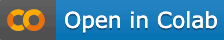# Budget allocation with Markov model### Import libraries​

``library(ChannelAttributionPro)``

``password="mypassword"``

``Data = read.csv("https://app.channelattribution.net/data/Data.csv",sep=",")print(Data)``

### Set the total budget you want to allocate​

``total_budget_new=100000``

### Set the percentage of the budget you want to reallocate from your previous allocation​

#### The parameter can be set in the range (0,1]. Setting it to 0.1 means that you will reallocate the 10% of your previous allocation. Drastic changes in your previous allocation are not suggested because the allocation algorithm is based on ROI and thus it is a local optimization algorithm. We suggest to reallocate a small percentage each time​

``perc_reall=0.1``

### For each channel indicate its marketing spend​

#### This parameter is optional. If you do not know the marketing spends you can set it to NULL​

``tab_costs=data.frame('channel'=c('alpha','iota','eta','beta','theta','lambda','epsilon','zeta','kappa','gamma','mi','delta'),'value'=c(41111.43,10387.21,23816.66,6743.46,1650.4,523.52,709.94,288.77,269.46,153.57,0.61,0.43))``

### Perform attribution with Markov model​

#### var_value and var_null are optional and can be set to NULL if you do not have this information​

``res_markov=markov_model(Data, var_path="path", var_conv="total_conversions", var_value="total_conversion_value", row_sep=";", cha_sep=">", password=password)``

### Perform budget allocation​

``res=markov_budget_allocation(res_markov,total_budget_new,tab_costs,perc_reall,min_perc_budget,server="app.channelattribution.net",password=password)print(res)``